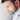Navigate back to the homepage

# Asymptotic computational complexity simplifiedBipin Paul Bedi
October 20th, 2018 · 2 min readThe asymptotic notation is the mathematical representation to analyze algorithm and represent its time (and/or space) complexity as its relation to input size. It describes its behavioral characteristics as the input size for the algorithm increases. The algorithm complexity can also be measured by monitoring time and space usage during its actual physical execution. This approach is not ideal for the following reasons

The accuracy and relativity (times obtained would only be relative to the machine they were computed on) of this method is bound to environmental variables such as computer hardware specifications, processing power, etc.
To conclude a general behavior we would have to execute it in several different scenarios.

Thus by representing an algorithm using asymptotic notation, it is much easier, faster and standard methodology to analyze and compare algorithms. For this post, we will restrict our discussion to time complexities {since the tremendous technological advancement has led to the cost of storage (persistent or ephemeral) as negligible}. In any problem domain, for a given algorithm f, with input size n we calculate some resultant runtime f(n). This results in a graph where the Y-axis is the runtime, X-axis is the input size, and plot points are the resultants of the amount of time for a given input size. We would mostly measure the worst-case scenario for any algorithm to compare different algorithms against the standard set of facts and dimentions.

Before analysing the algorithms, we shall establish certain common complexity classes in which an algorithm can be classified i.e. g(n) viz. K (or constant), log n, n, n*log n, n^2, n^3…, 2^n, 3^n…n^n

Types of Asymptotic Notation

Big-O
Big-O, commonly written as O, is an Asymptotic Notation for the worst case, or ceiling of growth for a given function. It provides us with an asymptotic upper bound for the growth rate of the runtime of an algorithm.
For e.g. f(n) is your algorithm runtime, and g(n) is an arbitrary time complexity you are trying to relate to your algorithm. f(n) is O(g(n)), if for some real constants c (c > 0) and n0, f(n) <= c g(n) for every input size n (n > n0)

`.css-1chxjt6{position:absolute;right:22px;top:24px;padding:8px 12px 7px;border-radius:5px;color:#6f7177;-webkit-transition:background 0.3s ease;transition:background 0.3s ease;}.css-1chxjt6:hover{background:rgba(255,255,255,0.07);}.css-1chxjt6[data-a11y="true"]:focus::after{content:"";position:absolute;left:-2%;top:-2%;width:104%;height:104%;border:2px solid var(--theme-ui-colors-accent,#6166DC);border-radius:5px;background:rgba(255,255,255,0.01);}@media (max-width:45.9375em){.css-1chxjt6{display:none;}}1f(n) = O(g(n) For K and N02if f(n) <= k * g(n) where n>=n03e.g.4f(n) = 2n^2 + 3n + 15since 2n^2 + 3n^2 + n2 = 6n^26f(n) = 2n^2 + 3n + 1 <= 6n^2 for n >= ?7f(n) <= k * g(n)8i.e. 6 * n^29Thus f(n) = O(n^2)`

Big-Omega
Big-Omega, commonly written as Ω, is an Asymptotic Notation for the best case, or a floor growth rate for a given function. It provides us with an asymptotic lower bound for the growth rate of the runtime of an algorithm.
f(n) is Ω(g(n)), if for some real constants c (c > 0) and n0 (n0 > 0), f(n) is >= c g(n) for every input size n (n > n0).

`1f(n) = BIG-OMEGA(g(n) For K and N02if f(n) >= k * g(n) where n>=n03e.g.4f(n) = 2n^2 + 3n + 15f(n) = 2n^2 + 3n + 1 >= n^2 for n >= ?6f(n) <= k * g(n)7i.e. 1 * n^28or k * g(n)9Thus f(n) = BIG-OMEGA(n^2)`

Theta
Theta, commonly written as Θ, is an Asymptotic Notation to denote the asymptotically tight bound on the growth rate of the runtime of an algorithm.
i.e. if O(g(n)) = Ω(g(n))
Then
f(n) = Θ(g(n))

`1If f(n) = O(n2)2and f(n) = BIG-OMEGA(n^2)3also4f(n) = O(g(n)) and f(n) = BIG-OMEGA(g(n))5Then f(n) = THETA(g(n))6Thus f(n) = THETA(n^2)`

Note The asymptotic growth rates provided by big-O and big-omega notation may or may not be asymptotically tight. Thus we use small-o and small-omega notation to denote bounds that are not asymptotically tight.

The main difference is that in f(n) = O(g(n)), the bound f(n) <= g(n) holds for some constant c > 0, but in f(n) = o(g(n)), the bound f(n) < c g(n) holds for all constants c > 0. Similarly f(n) = Ω(g(n)), the bound f(n) >= g(n) holds for some constant c > 0, but in f(n) = ω(g(n)), the bound f(n) > c g(n) holds for all constants c > 0.

`1Calculating for n!2if f(n) = n!3f(n) = n * (n-1) * (n-2)... 2 * 14For upper bound = n * n * n * n * n * n * n5i.e. f(n) = n! <= n^n for n>=?6f(n) = O(n^n)7For lower bound = 1 * 1 * 1 * 1 * 1...18= k9Thus f(n) = BIG-OMEGA(1) or BIG-OMEGA(K)10since O and BIG-OMEGA for n! is not equal it does not have a tight bound`

#biasing #algorithm #algorithm-design

### More articles from Bipin### L1 & L2 model regularizations techniques

feature engineering for machine learning models

October 12th, 2018 · 2 min read### Developers guide to designing REST endpoints

Coding blueprint for pragmatic rest api developers

October 10th, 2018 · 4 min read